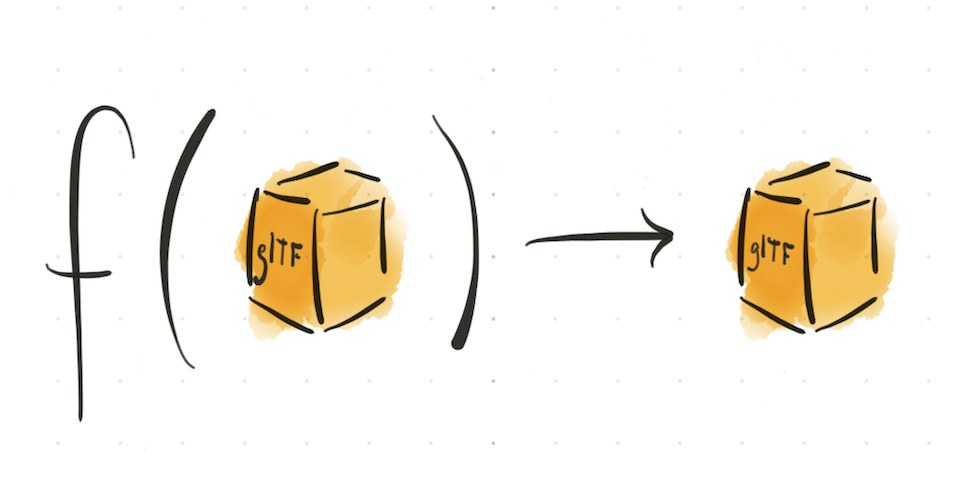# simplify

• Simplification algorithm, based on meshoptimizer, producing meshes with fewer triangles and vertices. Simplification is lossy, but the algorithm aims to preserve visual quality as much as possible for given parameters.

The algorithm aims to reach the target 'ratio', while minimizing error. If error exceeds the specified 'error' threshold, the algorithm will quit before reaching the target ratio. Examples:

• ratio=0.0, error=0.0001: Aims for maximum simplification, constrained to 0.01% error.
• ratio=0.5, error=0.0001: Aims for 50% simplification, constrained to 0.01% error.
• ratio=0.5, error=1: Aims for 50% simplification, unconstrained by error.

Topology, particularly split vertices, will also limit the simplifier. For best results, apply a weld operation before simplification.

Example:

``````import { simplify, weld } from '@gltf-transform/functions';
import { MeshoptSimplifier } from 'meshoptimizer';

await document.transform(
weld({ tolerance: 0.0001 }),
simplify({ simplifier: MeshoptSimplifier, ratio: 0.75, error: 0.001 })
);
``````

References:Made by Don McCurdy. Documentation built with greendoc and published under Creative Commons Attribution 3.0.